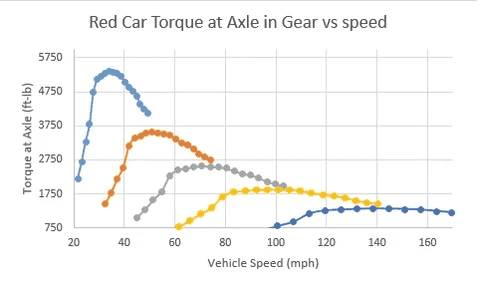# Gear ratios for this motorcycle

• i_am_imbact

#### i_am_imbact

Hello guys

I have a motorcycle engine in which I know the ratios of the transmission. and given the diagram of Power and rpm I made a function of P(ω) which is a polynomial of 6 order.

Then I said that F(friction)*u=P(ω) then I substitute ω=u*r/v v(ratio) and have F(u).Next knowing the Pmax and the ratios I found the speed in which we should change the gear 1st to 2nd and etc (Fu=Pmax) and in the end I have 5 equation of F (5 gears in the transmission).

In the end I tried to solve the first differential for the first gear (Fu-1/2*b*cd*u^2=m*du/dt) but there is no solution(tried to solve it with matlab).Do you know what I have to change or where I did a mistake in order to solve my problem?

Last edited by a moderator:

Maybe see if this website helps with your problem. It's looking at shift points from a practical point of view, using dynomometer measurements to back it all up. Maybe it will give you some insights into the way that you want to try to do this with calculations...

https://www.evodynamics.com/blogs/news/114447365-calculating-shift-points•FactChecker and Tom.G
From that nice graph that @berkeman found, it looks like the shift point is at or just before the intersection of the curves for adjacent gears.

Given that, subtract the equations of two adjacent gears and solve to find where they intersect.
f (1stGear) - f (2ndGear) = 0

Cheers,
Tom

•FactChecker
In the end I tried to solve the first differential for the first gear (Fu-1/2*b*cd*u^2=m*du/dt) but there is no solution(tried to solve it with matlab).
A solution for what unknown? What is it you want to find?

From what I understand (I assume you mean F(u) not Fu), you know F (which is a function of u) and you know b, cd & m. You then have a function of du/dt with respect to u. Knowing your velocity, you know your acceleration.

Welcome to PF.
If you have equations for 5 gears you can solve for the 4 equal power points for the gear changes.
I think you need to explain what you are trying to solve for.
It is often the case that clearly explaining the problem finds the answer.

From that nice graph that @berkeman found, it looks like the shift point is at or just before the intersection of the curves for adjacent gears.

Given that, subtract the equations of two adjacent gears and solve to find where they intersect.
f (1stGear) - f (2ndGear) = 0
The OP does not specify how the gear ratios were determined. From the example graph, it looks as though the shift point might be the RPM redline, which might be before the torque curves intersect. I suspect that will be true for any well-designed transmission gear ratios. Certainly that is true for the low gears of any motorcycle that I have ridden. I guess that the design of the upper gear ratios might be influenced by the typical highway cruising speed.

Last edited: